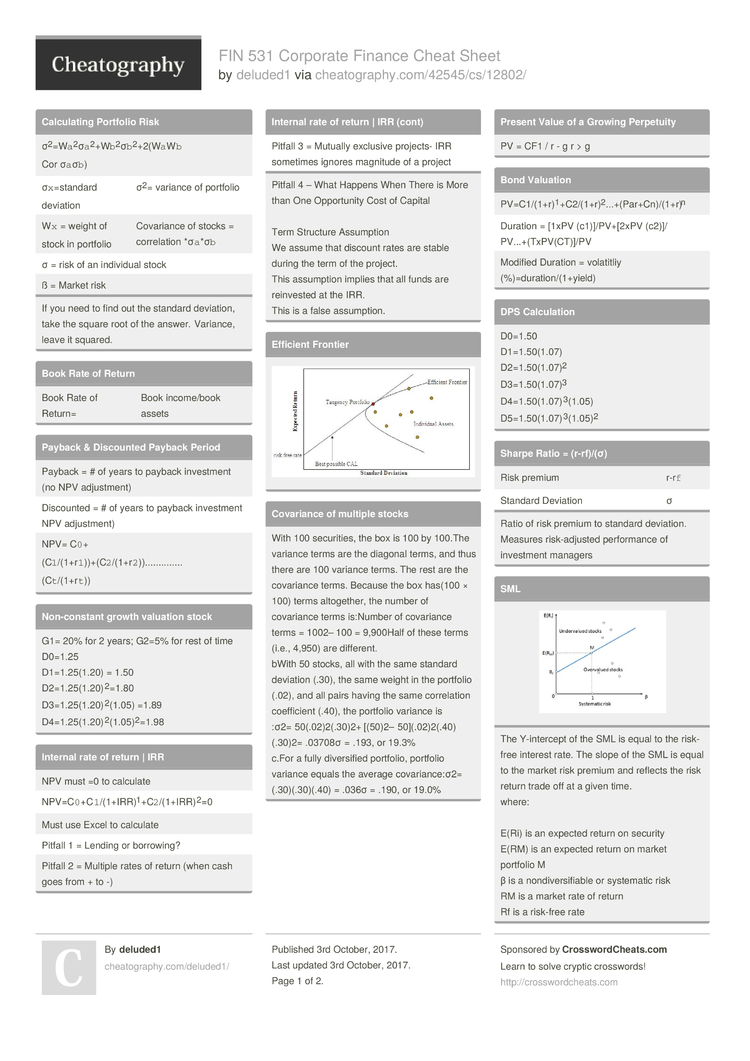# FIN 531 Corporate Finance Cheat Sheet by deluded1

FIN 531 Mid-Term Cheat Sheet

### Calcul­ating Portfolio Risk

 σ2=W­`a`­2­σ`a­`2­+W­`b`­2­σ`b­`2­+2­(W`­a`W`b` Cor σ`a`σ`b`) σ`x`=s­tandard deviation σ2= variance of portfolio W`x` = weight of stock in portfolio Covariance of stocks = correl­ation *σ`a`­*σ`b` σ = risk of an individual stock ß = Market risk
If you need to find out the standard deviation, take the square root of the answer. Variance, leave it squared.

### Book Rate of Return

 Book Rate of Return= Book income­/book assets

### Payback & Discounted Payback Period

 Payback = # of years to payback investment (no NPV adjust­ment) Discounted = # of years to payback investment NPV adjust­ment) NPV= C`0`+(­C`1­`/(­1+r­`1`­))+­(C`­2`/­(1+­r`2­`))..............(­C`t­`/(­1+r­`t`))

### Non-co­nstant growth valuation stock

 G1= 20% for 2 years; G2=5% for rest of time D0=1.25 D1=1.2­5(1.20) = 1.50 D2=1.2­5(1.20­)2­=1.80 D3=1.2­5(1.20­)2­(1.05) =1.89 D4=1.2­5(1.20­)2­(1.05­)2­=1.98

### Internal rate of return | IRR

 NPV must =0 to calculate NPV=C`­0`+­C`1­`/(­1+I­RR)­1­+C`­2`/­(1+­IRR­)2=0 Must use Excel to calculate Pitfall 1 = Lending or borrowing? Pitfall 2 = Multiple rates of return (when cash goes from + to -) Pitfall 3 = Mutually exclusive projects- IRR sometimes ignores magnitude of a project
Pitfall 4 – What Happens When There is More than One Opport­unity Cost of Capital

Term Structure Assumption
We assume that discount rates are stable during the term of the project.
This assumption implies that all funds are reinvested at the IRR.
This is a false assump­tion.

### Efficient Frontier### Covariance of multiple stocks

 With 100 securi­ties, the box is 100 by 100.The variance terms are the diagonal terms, and thus there are 100 variance terms. The rest are the covariance terms. Because the box has(100 × 100) terms altoge­ther, the number of covariance terms is:Number of covariance terms = 1002– 100 = 9,900Half of these terms (i.e., 4,950) are different. bWith 50 stocks, all with the same standard deviation (.30), the same weight in the portfolio (.02), and all pairs having the same correl­ation coeffi­cient (.40), the portfolio variance is :σ2= 50(.02­)2(.30)2+ [(50)2– 50](.0­2)2­(.4­0)(.30)2= .03708σ = .193, or 19.3% c.For a fully divers­ified portfolio, portfolio variance equals the average covari­anc­e:σ2= (.30)(.30­)(.40) = .036σ = .190, or 19.0%

### Present Value of a Growing Perpetuity

 PV = CF1 / r - g r > g

### Bond Valuation

 PV=C1/­(1+­r)­1+­C2/­(1+­r)­2...+­(Pa­r+C­n)/­(1+­r)n Duration = [1xPV (c1)]/­PV+­[2xPV (c2)]/ PV...+­(Tx­PV(­CT)]/PV Modified Duration = volatitliy (%)=du­rat­ion­/(1­+yield)

### DPS Calcul­ation

 D0=1.50 D1=1.5­0(1.07) D2=1.5­0(1.07)2 D3=1.5­0(1.07)3 D4=1.5­0(1.07­)3­(1.05) D5=1.5­0(1.07­)3­(1.05)2

### Sharpe Ratio = (r-rf­)/(σ)

 Risk premium r-r`f` Standard Deviation σ
Ratio of risk premium to standard deviation. Measures risk-a­djusted perfor­mance of investment managers

### SMLThe Y-inte­rcept of the SML is equal to the risk-free interest rate. The slope of the SML is equal to the market risk premium and reflects the risk return trade off at a given time.
where:

E(Ri) is an expected return on security
E(RM) is an expected return on market portfolio M
β is a nondiv­ers­ifiable or systematic risk
RM is a market rate of return
Rf is a risk-free rate

### Efficient Frontier

 The efficient frontier is the set of optimal portfolios that offers the highest expected return for a defined level of risk or the lowest risk for a given level of expected return. Portfolios that lie below the efficient frontier are sub-op­timal, because they do not provide enough return for the level of risk.

### Stock Returns

 R=(P`t­+1`­-P`­t`+­D)/­(P`t`) R= Return P`t`= Stock Price @ time D = Dividend

### CAPM = Capital Asset Pricing Model

 r`i`=r­`f`­+ß(­r`m­`-r`f`) ß=Beta r`f`= risk free rate r`m` = market rate i = investment E(r) = expected return Expected risk premium on stock= beta x expected risk premium on market r-r`f`­=ß(­r`m­`-r`f`)
Beta is the extent to which a stock moves with the market. CAPM says that the higher the Beta, the higher the risk

### Market Efficiency

 1.) Weak = Market reflects past info 2.) Semi-S­trong = Past & Current public info is reflected 3.) All inform­ation is reflected in the stock price (public & private)
Efficient Market - Market in which inform­ation is reflected in stock prices quickly + correctly

### Profit­ability Index

 PI = NPV/In­ves­tment
Another Example - continued
Proj NPV Investment PI
A 230,000 200,000 1.15
B 141,250 125,000 1.13
C 194,250 175,000 1.11
D 162,000 150,000 1.08

Select projects with highest Weighted Avg PI
WAPI (BD) = 1.13(125) + 1.08(150) + 0.0 (25)
(300) (300) (300)
= 1.01

### Captal Rationing

 Capital Rationing - Limit set on the amount of funds available for invest­ment. Soft Rationing - Limits on available funds imposed by manage­ment. Hard Rationing - Limits on available funds imposed by the unavai­lab­ility of funds in the capital market.

 =r`m`-r`f`
For any invest­ment, we can find the opport­unity cost of capital using the security market line. With  = 0.8, the opport­unity cost of capital is:
r = rf + B(rm – rf)
r = 0.04 + [0.8 B (0.12 – 0.04)] = 0.104 = 10.4%

The opport­unity cost of capital is 10.4% and the investment is expected to earn 9.8%. Therefore, the investment has a negative NPV.

If return is 11.2% What is Beta?
r = rf + (rm – rf)
0.112 = 0.04 + (0.12 – 0.04)   = 0.92 Pages
//media.cheatography.com/storage/thumb/deluded1_fin-531-corporate-finance.750.jpg

PDF (recommended)